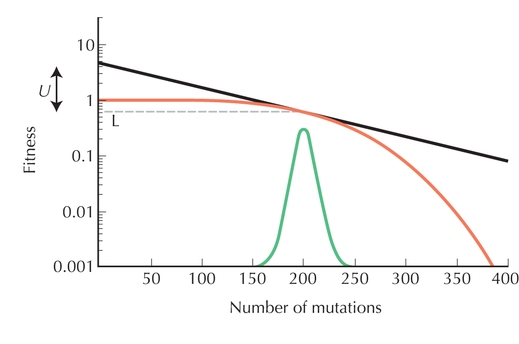Figure P23.6. (Upper curve) How fitness decreases with the number of deleterious mutations, as in Figure P23.2. (Bell-shaped curve) Distribution of the number of deleterious mutations at equilibrium with U = 2. (Dashed line) Equilibrium mean fitness, at exp(–0.5) = 0.61; (solid line) tangent to the upper curve, and so its slope gives the selection coefficient against deleterious mutations, s = 0.01. Because the mean number of deleterious mutations is U/s, the distance between the dashed line and the intercept of the tangent equals U, which here equals 2. (The vertical scale is logarithmic, and so the distance between 0.1 and 1 [say] is loge(10) = 2.30.)